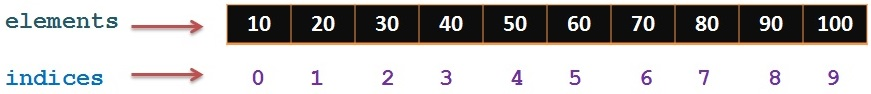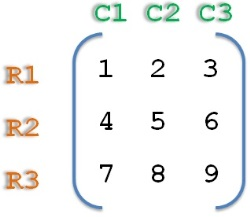# Array in Java

An `array `is a collection of similar data types.

It contains the values of homogenous or similar type.

It is also known as static data structure because size of an array must be specified at the time of its declaration.

It can contain values of either primitive or reference type.

It gets memory in heap area.

Index of array starts from 0 to array_size – 1.In the above diagram, we are representing one array with its indices.

First index starts at 0(zero)

Its size is 10 and hence last index of this array is 10 -1 (`size -1`) = 9 and element is 100 at the last index.

##### What are the features of Array?

It’s a collection of similar data types and hence we can store only similar type of elements.

We can store only fixed set of elements as its size is `static` and it will not grow dynamically.

It occupies a contiguous memory location

It is indexed data type and index begins from 0 to size -1

We can access any element using its index

We can easily perform sort and search on the Array.

##### Array declaration syntax

1. Datatype[] identifier;
2. Datatype  identifier[];
```Datatype[] identifier;
Datatype  identifier[];```

###### Examples:

1. int[ ] arr;
2. char[ ] arr;
3. float[ ] arr;
4. long[ ] arr;
5. int[ ][ ] arr;   // two dimensional array.
```int[ ] arr;
char[ ] arr;
float[ ] arr;
long[ ] arr;
int[ ][ ] arr;   // two dimensional array.```

##### Initialization of Array

###### Array can be initialized using one of the 2 ways

1.Using new operator

2. Using array initialization block

###### 1.Using new operator

In this case, we will use “new” operator to create the object of an array

Example:

1. int marks[] = new int;
`int marks[] = new int;`

The above line creates an empty array named “`marks`” of integer type whose size is 5.

1. char alphabets[] = new char;
`char alphabets[] = new char;`

The above line creates an empty array named “`alphabets`” of character type whose size is 26.

###### 2. Using array initialization block

In this case, we will directly assign the values to the array

Example:

1. int marks[] = {90,45,80,75,100};
`int marks[] = {90,45,80,75,100};`

##### Accessing array element

We can access the element in the array using its index.

As we know that index starts from 0 to size -1, to access first element of array, we can use `arrayName`

We can access last element of array using `arrayName[size-1]`

We can access 5th element using `arrayName.`

###### Example:

`marks` gives 90, `marks` gives 80 and `marks` gives 100.

Length or size of an Array can be found using `arrayName.length`

Where ‘length’ is the variable name.

##### How to iterate array using traditional for loop

1. package com.kb.arrays;
2.
4.
5.     public static void main(String[] args) {
6.         int marks[] = {90,45,80,75,100};
7.         for(int index=0;index<marks.length;index++){
8.             System.out.println("Array element is "+marks[index]);
9.         }
10.
11.     }
12.
13. }
```package com.kb.arrays;

public static void main(String[] args) {
int marks[] = {90,45,80,75,100};
for(int index=0;index<marks.length;index++){
System.out.println("Array element is "+marks[index]);
}

}

}```

##### How to iterate array using enhanced for loop

1. package com.kb.arrays;
2.
3. public class EnhancedForLoop {
4.
5.     public static void main(String[] args) {
6.         int marks[] = { 90, 45, 80, 75, 100 };
7.         for (int element : marks) {
8.             System.out.println("Array element is " + element);
9.         }
10.     }
11.
12. }
```package com.kb.arrays;

public class EnhancedForLoop {

public static void main(String[] args) {
int marks[] = { 90, 45, 80, 75, 100 };
for (int element : marks) {
System.out.println("Array element is " + element);
}
}

}```

##### Multidimensional Arrays in Java

So far, we have seen single dimensional arrays where it will have single row and multiple columns.

Each element is present in one column in a single row and hence its single dimensional array.

In case of multidimensional array,elements are arranged in multiple rows and multiple columns.###### Its syntax is

1. datatype[][] identifier;
`datatype[][] identifier; `

or

1. datatype identifier[][];
`datatype identifier[][];`

##### Initialization of Multidimensional Array

###### Multidimensional Array can also be initialized using one of the 2 ways

1.Using new operator

2. Using array initialization block

###### 1.Using new operator

In this case, we will use “new” operator to create the object of an array

Example:

1. int matrix[][] = new int;
`int matrix[][] = new int;`

The above line creates an empty 2 dimensional array named “`matrix`” of integer type whose size is 9(`3*3`) which means 3 rows and 3 columns

###### 2. Using array initialization block

In this case, we will directly assign the values to the array

Example:

1. int matrix[][] = {{1,2,3},{4,5,6},{7,8,9}};
`int matrix[][] = {{1,2,3},{4,5,6},{7,8,9}};`

##### How to access elements in 2 dimensional array

Index of both row and column starts with 0(zero)So, accessing element at 2nd row and 3rd column is `Matrix` and which is “6”.

Element at 3rd row and 2nd column is `Matrix` which is “8”.

In the above matrix 2 dimensional array, row size is 3 and column size is also 3 and hence its `3*3` matrix

Accessing last element in the above matrix is `matrix[row-1][column-1]`

Which is `Matrix[3-1][3-1] = matrix` and which is “9”.